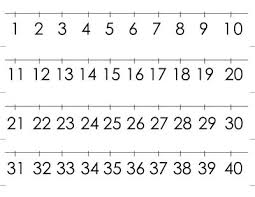# What is 16

What is the sum of three consecutive even integers such that six more than twice the second is 2/3 of the first increased by 3/2 of the third?Did you find an error or inaccuracy? Feel free to write us. Thank you!Tips to related online calculators
Need help to calculate sum, simplify or multiply fractions? Try our fraction calculator.
Do you have a system of equations and looking for calculator system of linear equations?